## Colored Triangular Tetriamonds

As already mentioned, matching rules for triangular tetriamonds using n colors are different from those for single triangles with n^2 colors. Nevertheless the numbers of one-sided pieces are equal in both cases. For two-sided pieces we get different counts because the combinations of colors at the three sides of a triangular tetriamond are reversed in order if the piece is flipped, whereas the colors of the single triangle don't change. The following example with rr = 1, rg = 2, gr = 3, gg = 4 shows the difference.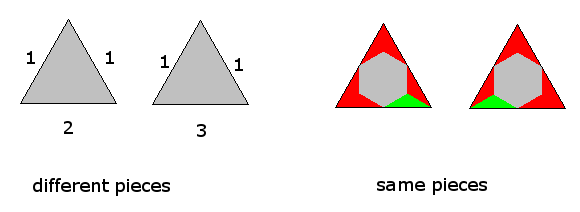Click the numbers of pieces to get the constructions with different sets.

Colors Flip Type Number of Pieces Total Number
of Triangles
Constructions
n one-sided (n^6+2n^2)/3 (n^6+2n^2)/3*4
two-sided (n^6+3n^3+2n^2)/6 (n^6+3n^3+2n^2)/6*4
2 one-sided 24 96 triangle, parallelogram, trapezium
two-sided 16 64 triangle, truncated rhomb
3 one-sided 249 996 semiregular hexagon
two-sided 138 552 holey hexagon, holey triangle, berry

### Two Colors, one-sided

The 24 single triangles with 4 colors can fill a hexagon with side length 2. With the corresponding 24 triangular tetriamonds a hexagon with side length 4 can also be obtained but the construction is different.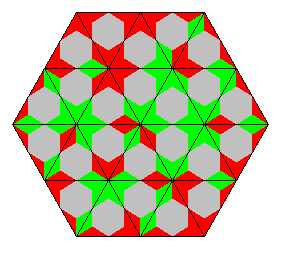A solution for a parallelogram and a trapezium is also possible.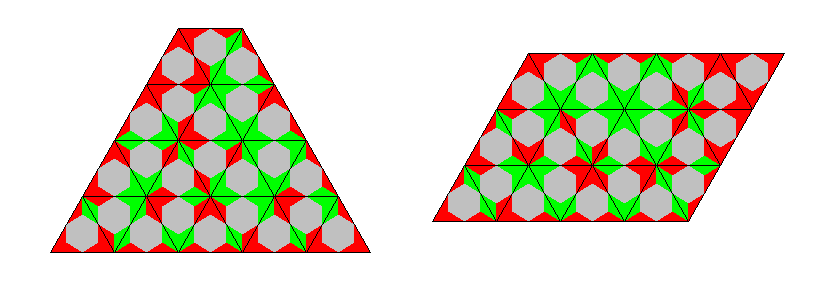### Two Colors, two-sided

There are 16 pieces and for each color there are 13 same colored edges. We can't get a figure with an uniformly colored border because for the other color the number of same colored edges is odd and a match for these edges isn't possible. Instead you can take for each color an odd number of same colored edges and place them at the border. At the triangle you can see 11 green edges and 1 red edge and at the hexagon 5 edges of each color.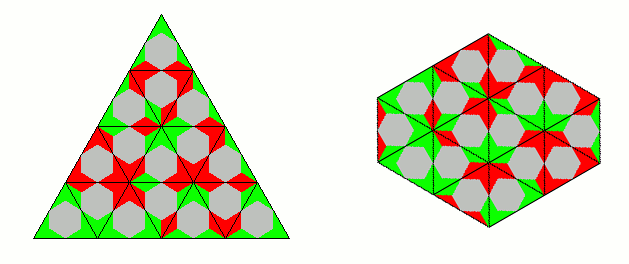### Three Colors, one-sided

Among the 249 pieces there are 249*3/9 = 83 same colored edges for each color. An odd number of them must be at the border to get an even number of them to match in the interior of the figure. For the edges with color combinations there is no problem, because r-g matches with g-r and so on.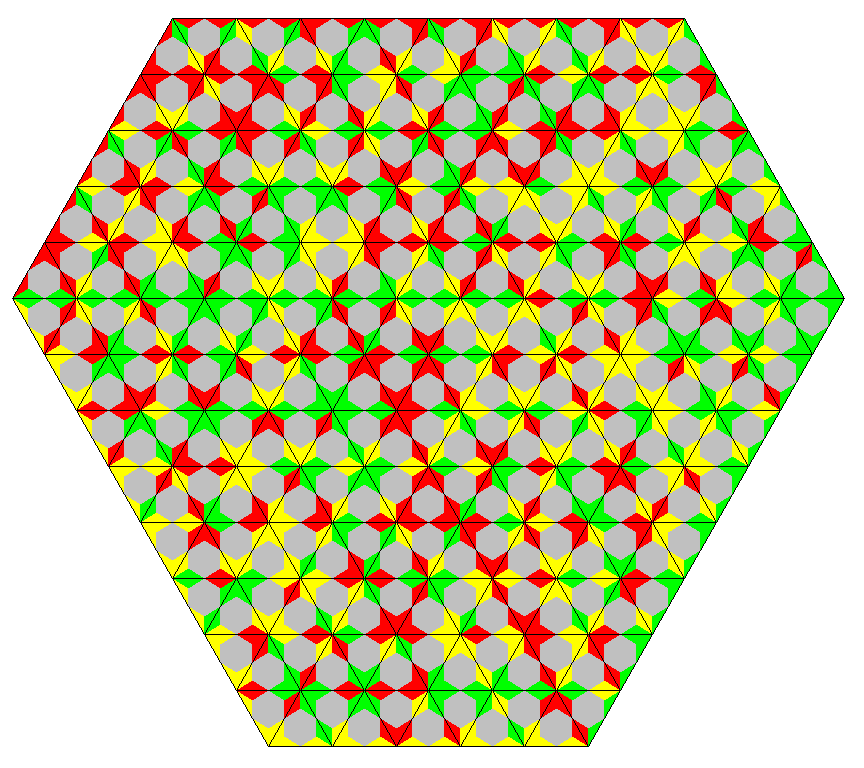### Three Colors, two-sided

There are 138 pieces. This is enough for a triangle of size 24 with an hexagonal hole of size 2 at the center. The picture shows that the number of each edge type is odd. For the same colored edges we have 24+1+2k. Therefore a figure with no hole and an uniformly colored border isn't possible.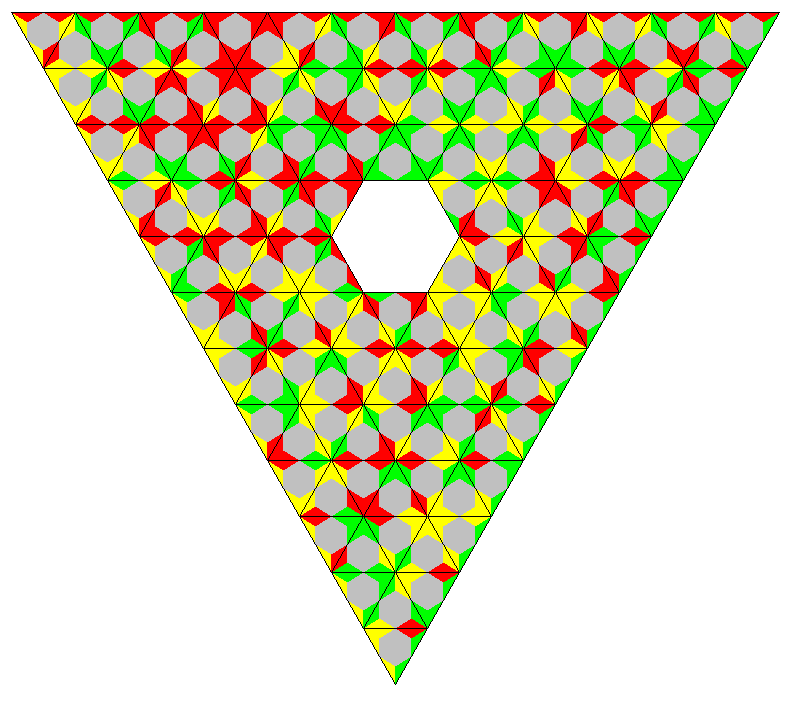The following construction has an uniformly colored outer border but this doesn't hold for the star like hole.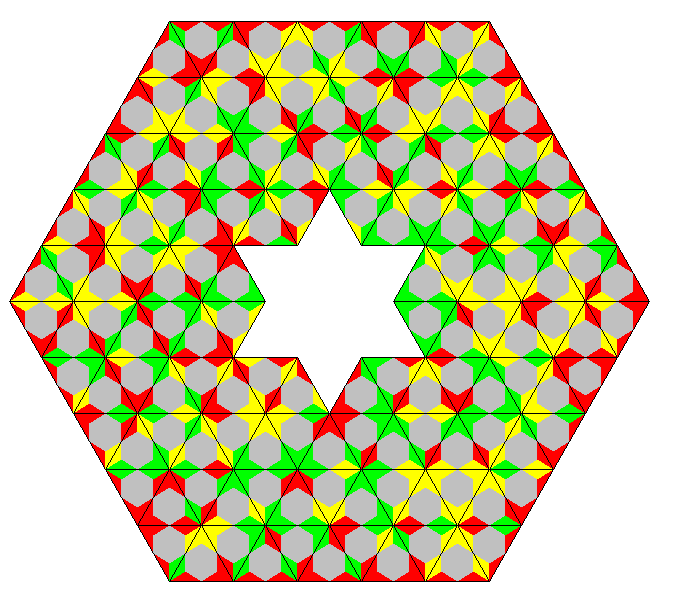If there is no hole the border must get an odd number of each edge type.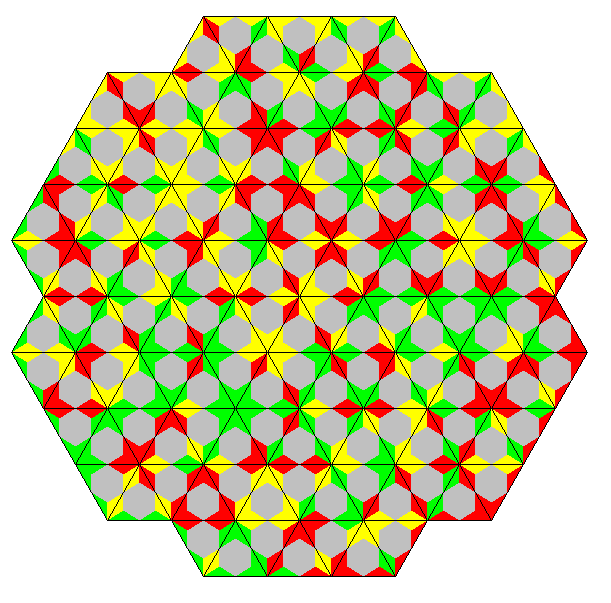Back
Home